### IMO Shortlist 2013 problem G2

Kvaliteta:
Avg: 3,0
Težina:
Avg: 6,0
Let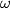$\omega$ be the circumcircle of a triangle$ABC$. Denote by$M$ and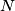$N$ the midpoints of the sides$AB$ and$AC$, respectively, and denote by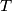$T$ the midpoint of the arc$BC$ of$\omega$ not containing$A$. The circumcircles of the triangles$AMT$ and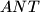$ANT$ intersect the perpendicular bisectors of$AC$ and$AB$ at points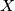$X$ and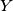$Y$, respectively; assume that$X$ and$Y$ lie inside the triangle$ABC$. The lines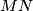$MN$ and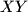$XY$ intersect at$K$. Prove that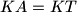$KA = KT$.
Izvor: Iran